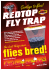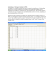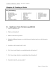# Genetics of Organisms

## Transcription

Genetics of Organisms
```AP Biology Lab
Name: _____________________________
Genetics of Organisms
Date: _________
Per: _________
Objectives
Before doing this lab you should understand:
Chi-square analysis of data & the life cycle of diploid organisms useful in genetics studies.
After doing this lab you should be able to:
Investigate the independent assortment of two genes and determine whether the two genes are
autosomal or sex-linked using a multigenerational experiment, and analyze the data from your
genetic crosses using chi-square analysis techniques.
Part A. Statistical Analysis Section
Example 1
Statistics can be used to determine if differences among groups are significant, or simply the
result of predictable error. The statistical test most frequently used to determine whether data
obtained experimentally provide a good fit or approximation to the expected or theoretical data is the
chi-square test. This test can be used to determine if deviations from the expected values are due to
chance alone, or to some other circumstance. For example, consider corn seedlings resulting from an
F1 cross between parents that are heterozygous for color.
A Punnett square of the F1 cross Gg x Gg would predict the expected proportion of green:albino
seedlings would be 3:1. Use this information to fill in the Expected (e) column and the (o-e) column in
Table 7.1.
Table 7.1
Phenotype
Green
Albino
Genotype
GG or Gg
gg
Total:
Observed (o)
72
12
84
Expected (e)
(o-e)
There is a small difference between the observed and expected results, but are these data close enough
that the difference can be explained by random chance or variation in the sample?
To determine if the observed data fall within the acceptable limits, a chi-square analysis is performed
to test the validity of a null hypothesis (that there is no statistically significant difference between the
observed and expected data). If the chi-square analysis indicates that the data vary too much from the
expected 3:1, an alternative hypothesis is accepted.
The formula for chi-square is
χ2 = Σ (o-e)2
e
where o = observed number of individuals
e = expected number of individuals
Σ = the sum of values (in this case the differences, squared, divided by the number of expected)
1. This statistical test will examine the null hypothesis, which predicts that the data from the
experimental cross above will be expected to fit the 3:1 ratio.
2. Use the data from Table 7.1 to complete table 7.2.
Table 7.2
Phenotype
Green
Albino
Observed (o)
Expected (e)
(o-e)
(o-e)2
72
12
χ2 =Σ (o-e)2
e
(o-e)2
e
Critical Values Table
Probability (p)
0.05
0.01
0.001
1
3.84
6.64
10.8
2
5.99
9.21
13.8
Degrees of Freedom (df)
3
7.82
11.3
16.3
4
9.49
13.2
18.5
5
11.1
15.1
20.5
How to use the Critical Values Table
1. Determine the degrees of freedom (df) for your experiment. It is the number of phenotypic classes
minus 1. Since there are two possible phenotypes, for this experiment df=1 (two samples -1). If this
experiment had gathered data for a dihybrid cross, there would be four possible phenotypes and
therefore 3 degrees of freedom.
2. Find the p value. Under the 1 df column, find the critical value in the probability (p) = 0.05 row; it
is 3.84. What does this mean? If the calculated chi-square value is greater than or equal to the
critical value from the table, then the null hypothesis is rejected. Since in our example χ2 =
5.14 and 5.14 > 3.84, we reject our null hypothesis that there is no statistically significant
difference between the observed and expected data. In other words, chance alone cannot explain
the deviations we observed and there is, therefore, a reason to doubt our original hypothesis (or to
question our data collection accuracy.) The minimum probability for rejecting a null hypothesis is
generally 0.05, so this is the row to use in s chi-square table.
3. These results are said to be significant at a probability of p = 0.05. This means that only 5% of the
time would you expect to see similar data if the null hypothesis was correct; thus, you are 95%
sure that the data did not fit a 3:1 ratio.
4. Since these data do not fit the expected 3:1 ratio, you must consider reasons for this variation.
Additional experimentation would be necessary. Perhaps the sample size was too small, or errors
were made in data collection. In this example, perhaps, the albino seedlings are underrepresented
because they died before the counting was performed.
Example 2
In a study of incomplete dominance in tobacco seedlings, the counts in table 7.3 were made from a
cross between two heterozygous plants.
Table 7.3
Phenotype
Green
Yellow Green
Albino
Observed (o)
Expected (e)
(o-e)
(o-e)2
(o-e)2
e
22
50
12
χ2 =Σ (o-e)2
e
A Punnett square for this cross indicates an expected ratio of 1 green:2 yellow green:1 albino.
Calculate the expected for each and fill out the Table 7.3.
How many degrees of freedom are there? _______
Using the critical values chart, what is the probability value for these data? _____
According to the critical value of χ2, can you accept or reject the null hypothesis (does the data fit the
expected 1:2:1 ratio?)
Practice Problem
An investigator observes that when pure-breeding, long-winged fruit flies are mated with purebreeding, short-winged flies, the F1 offspring have an intermediate wing length. When several
intermediate-winged flies are allowed to interbreed, the following results are obtained:
230 long-winged flies, 510 intermediate winged flies, 260 short winged flies.
a. What is the genotype of the F1 intermediate-wing-length flies?
b. Write a hypothesis describing the mode of inheritance of wing length in fruit flies (this is your null
hypothesis).
c. Complete Table 7.4
Table 7.4
Phenotype
Observed (o)
Expected (e)
(o-e)
(o-e)2
χ2 =Σ (o-e)2
e
d. How many degrees of freedom (df) are there? _____
e. Chi-square (χ2) = _____
f. What is the probability value for these data? _____
g. Can you accept or reject the null hypothesis? Explain why.
(o-e)2
e
Part B. Fruit Fly Crosses
Procedure 1: Monohybrid Cross
1. Go to the website: http://www.sciencecourseware.com/vcise/drosophila/ and log in as a guest.
2. “Order” a pair of flies: a male wild type for wing size and a female with vestigial wing size. Put them
in your “Shopping Cart”. Check out.
3. Breed the flies, click on the “Mating Jar” and record the Data in Table 7.5.
Table 7.5-F1 Generation
Phenotype and Symbol
Females
Males
4. Add one female and one male from the F1 Generation and put them into a new mating jar. Breed
them and record the F2 generation data in Table 7.6
Table 7.6-F2 Generation
Phenotype and Symbol
Females
Males
5. Write a hypothesis that describes the mode of inheritance of the trait you studied. This is your null
hypothesis.
6. Construct Punnett squares to predict the expected results of both parental and F1 generational
Parental Cross
F1 Cross
7. Refer to the Punnett squares above. In the table below, record the expected ratios for the genotypes
and phenotypes of the F1 and F2 crosses in the experiment.
Expected Genotypic Ratio
F1 generation
F2 generation
8. From the results, describe your cross.
Is the mutation sex-linked or autosomal?
Is the mutation dominant or recessive?
Is the cross monohybrid of dihybrid?
_________________
_________________
_________________
Expected Phenotypic Ratio
9. Perform a chi-square test on your F2 results to see if the deviations are within limits expected by
chance.
Phenotype
Observed (o)
Expected (e)
(o-e)
(o-e)2
(o-e)2
e
χ2 =Σ (o-e)2
e
10. Calculate the chi-square value for these data.
How many degrees of freedom are there?
Chi-square (X2)
What is the probability for this data?
11. Can you accept or reject your null hypothesis? Explain why.
Procedure 2: Monohybrid Cross
1. Return to the lab and click the “trash” to clear the lab of flies.
2. “Order” a pair of flies: a male wild type and a female with a yellow body. Check out
3. Breed the flies, click on the “Mating Jar” and record the Data in Table 7.7.
Table 7.7-F1 Generation
Phenotype and Symbol
Females
Males
4. Add one female and one male from the F1 Generation and put them into a new mating jar. Breed
them and record the F2 generation data in Table 7.8
Table 7.8-F2 Generation
Phenotype and Symbol
Females
Males
5. Write a null hypothesis that describes the mode of inheritance of the trait you studied.
6. Construct Punnett squares to predict the expected results of both parental and F1 generational
Parental Cross
F1 Cross
7. Refer to the Punnett squares above. In the table below, record the expected ratios for the genotypes
and phenotypes of the F1 and F2 crosses in the experiment.
Expected Genotypic Ratio
Expected Phenotypic Ratio
F1 generation
F2 generation
8. From the results, describe your cross.
Is the mutation sex-linked or autosomal?
Is the mutation dominant or recessive?
_________________
_________________
9. Perform a chi-square test on your F2 results to see if the deviations are within limits expected by
chance.
Phenotype
Observed (o)
Expected (e)
(o-e)
(o-e)2
χ2 =Σ (o-e)2
e
10. Calculate the chi-square value for these data.
How many degrees of freedom are there? _____
Chi-square (χ2) = _____
What is the probability for this data? _____
11. Can you accept or reject your null hypothesis? Explain why.
(o-e)2
e
Procedure 3: Dihybrid Cross
1. Return to the lab and click the “trash” to clear the lab of flies.
2. Order a wild type female and order a male with sepia eyes and black body.
3. Breed the flies, click on the “Mating Jar” and record the Data in Table 7.9.
Table 7.9-F1 Generation
Phenotype and Symbol
Females
Males
4. Add one female and one male from the F1 Generation and put them into a new mating jar. Breed
them and record the F2 generation data in Table 7.10
Table 7.10-F2 Generation
Phenotype and Symbol
Females
Males
5. Write a null hypothesis that describes the mode of inheritance of the traits you studied.
6. Construct Punnett squares to predict the expected results of both parental and F1 generational
Parental Cross
F1 Cross
7. Refer to the Punnett squares above. In the table below, record the expected ratios for the genotypes
and phenotypes of the F1 and F2 crosses in the experiment.
Expected Genotypic Ratio
F1 generation
F2 generation
8. From the results, describe your cross.
Are the mutations sex-linked or autosomal? _________________
Are the mutations dominant or recessive? _________________
Is the cross monohybrid of dihybrid?
_________________
Expected Phenotypic Ratio
9. Perform a chi-square test on your F2 results to see if the deviations are within limits expected by
chance.
Phenotype
Observed (o)
Expected (e)
(o-e)
(o-e)2
χ2 =Σ (o-e)2
e
10. Calculate the chi-square value for these data.
How many degrees of freedom are there? _____
Chi-square (χ2) = _____
What is the probability for this data? _____
11. Can you accept or reject your null hypothesis? Explain why.
(o-e)2
e
```

### Chi square test of Froot Loops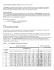### Staff Council Newsletter, 2014 Staff Council Meets with BOT and President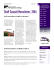### BrunerG - CIBMTR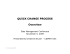### Catching flies game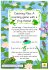### Red Top Fly Trap